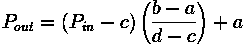# Contrast Stretching or Contrast Normalization an Image in MATLAB

Contrast stretching is a simple image enhancement technique that improves the contrast in an image by expanding the dynamic range of intensity values it contains. It can only apply a linear scaling function to the image pixel values.

## Some Mathematical Modelling: (source: ed.ac.uk)

It is necessary to specify the upper and lower pixel value limits over which the image is to be normalized. Often these limits will just be the minimum and maximum pixel values that the image type concerned allows. For example for 8-bit graylevel images the lower & upper limits might be 0 and 255. Call the lower and the upper limits a and b respectively.
The simplest of stretching scans the image to find the lowest & highest pixel values in the image. Call these c and d. Then each pixel P is scaled using the following function:Values below 0 are set to 0 and values about 255 are set to 255.

MATLAB implementation of the above concept of Contrast Stretching or Contrast Normalization on an image is:
figure(1);
imshow(X);
title('Original Image')
a = min(X(:));
%minimum pixel of image X
b = max(X(:));
%maximum pixel of image X
X= (X-a).*(255/(b-a));
%just using the formula above
figure(2);
imshow(X);
title('Contrast Streached Image')

Results of Contrast Stretching in MATLAB:The original Image: Not Contrast StretchedThe Resultant Image: Contrast Stretched